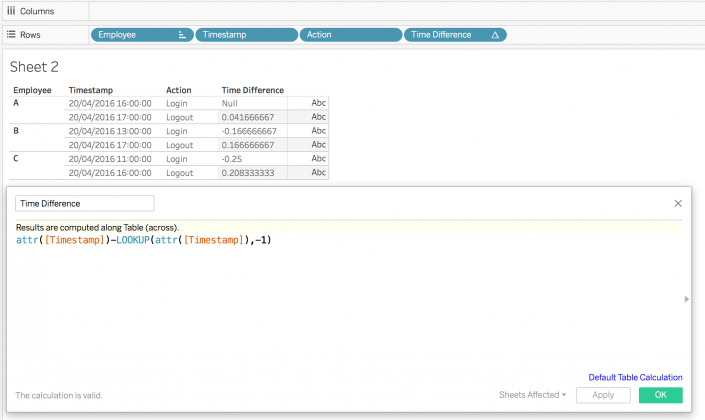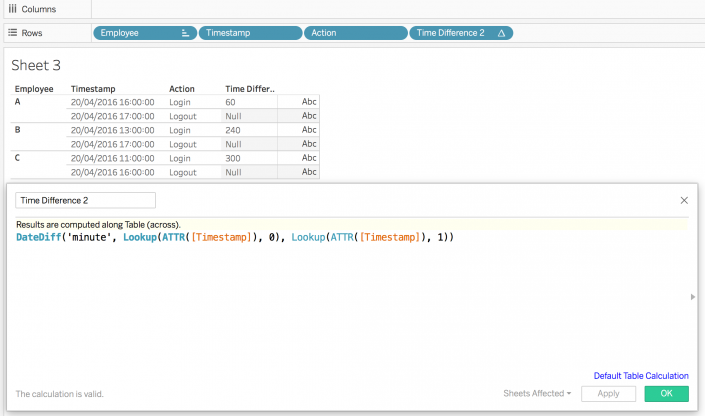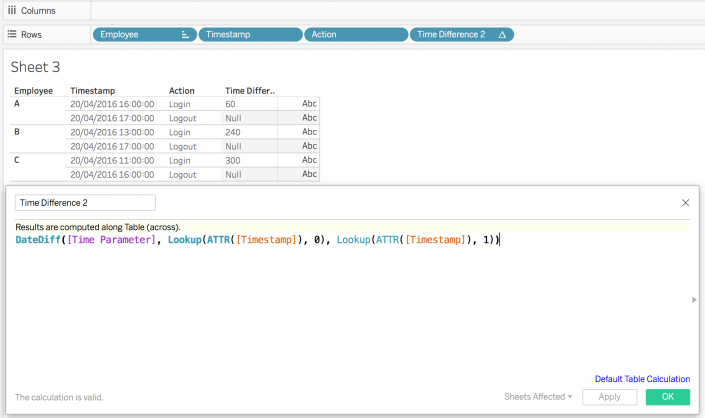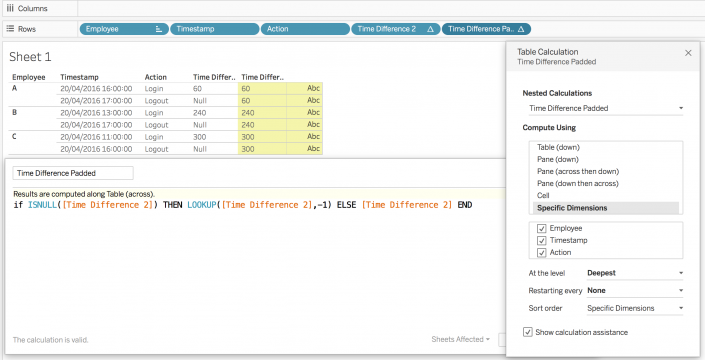## Calculate DateDiff with One Column in Tableau

While manning our support desk you sometimes get some really good Tableau related challenges from your clients. One of the tickets that came in recently was related to calculating the time difference between when people login and people log out of a system. However, and here comes the challenges, we only have one date or timestamp column – logging in and out is defined by a dimension.

My initial thought was to create two IF-calculations along the lines of when the dimension is ‘login’ give me a timestamp and another calculation that does the same for ‘logout’. However, and this is quite logic, this will not work. You will have null values in each column of these calculations. Hence Tableau’s datediff function will not work (it will basically result in nulls).

I went back to the client and suggested to reshuffle the data and create two date columns. One with the timestamp for login and one for when they logout – which would then make the datediff calculation fairly easy. But this wasn’t a feasible solution for the client and so I went back to finding a solution for one date column. Fortunately, the Tableau Community forums are a great resource for problems like this. I found an old thread in which someone had a similar problem and the solution was using table calculations to lookup the two date values you need to pass in the datediff calculation.

With the lookup function we can find values in different rows. For example, lookup([Sales],-1) will give you the sales value of the row above the current row (row 0). If we apply this logic to our timestamp column we can find the data in row+1 (the logout row) and detract that from the login row (row 0).

I created a dummy dataset for this blog. It has one column called timestamp and a flag with the action (either login or logout). I first started out with this calculation:However, this doesn’t calculate the time difference correctly. We’ll need Tableau’s datediff calculation to actually calculate on the correct level (minutes).What I’m doing here is lookup the current row (0) and then do a datediff with the next row (row +1). The unit of date is minutes in this calculation, however, you can also create a parameter that switches between minutes and hours. Just replace the ‘minute’ with your string parameter.In order to remove the null values you can either replace them with zeros with a zn() calculation or return the value from the next row.Many people consider Tableau’s table calculations a mystery, but I hope I demystified the lookup function in this blog. Please reach out on Twitter or in the comment section below in case you have a problem or you need more information.

London, UK

### 10 thoughts on “Calculate DateDiff with One Column in Tableau”

1.Jay Lewis says:

If you had something akin to a Session_ID (as opposed to just employee number), then a single LOD calc could work well, too.

{FIXED Session_ID : DATEDIFF([TimeParameter],MIN(Timestamp),MAX(Timestamp)}

I lean heavily on LODs in web analytics for calculations, and it’s a similar challenge where all the timestamps are in one column. The basic structure in clickstream data is [Session_ID, Page, Timestamp]. The calc above would give me session duration, but slightly different LODs can compute metrics like sessions per day and pageviews per session.

1.Andre De Vries says:

Yes, a single LOD would also work. This one for example:

DATEDIFF(‘minute’,{FIXED [Employee]: min((IF [Action] = ‘Login’ THEN [Timestamp] END))},{FIXED [Employee]: min((IF [Action] = ‘Logout’ THEN [Timestamp] END))})

But I wanted to highlight the lookup Table Calculation. Many people still struggle with table calculations in Tableau and I hope this blog helped them understand it a little bit more.

2.Robbert Korthals says:

Great discussion going on here!

I had the following question though…

How would you go about defining SessionIDs per consumer as dimensions (ie not a measure) directly in Tableau?

3.Robbert Korthals says:

I would like to group timestamps with a session ID based on the time difference with the previous timestamp.

eg. if there is a 10 minute difference compared to the last timestamp, I want to start a new Session ID (ie jump from SessionID 1 to SessionID 2).

Any idea how to tackle this?

1.Tony Roberts says:

Ever figure this out? I’m trying to create some sort of Run_ID or session_ID

4.Harish says:

Hey Andre,

Nice blog, very informative 🙂

Is it possible to use a “Lookup Function” when I want the difference in a slightly different method, the difference should be calculated by having the first date as the base date?

For example : date diff: row(1) – row(0)
row(2) – row (0)

Thanks,

Harish

1.Andre De Vries says:

Hello Harish,

Yes, you could change the calculation to not look at 0 first, but at 1 – so one row ahead and then one row back.

So basically swapping the calculation around in my example.

5.Lisa says:

Hi Andre,
this is a very nice explaination. But what I find challenging with Table Calculation is, that they are only valid within the Canvas. How would i visualize this result? E.g. if I wanted to create a line chart with average time spent logged on per day?

Thanks
Lisa

6.Tejas says:

Hi Andre,

Great Trick. But would it not be easy to unpivot the table with Timestamp and dimension column to create 2 separate columns for Login time and Logout time.

That would have made it simple to calculate the datediff.

7.Luke says:

Hi Andre
I tried to find a solution to Calculate the time difference for so long, and this’s just what I need, thanks for sharing your idea!